# honey jose presentation

8 Apr 2016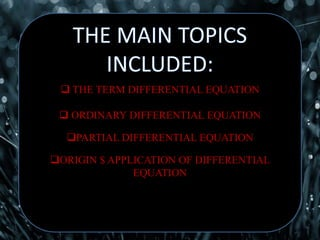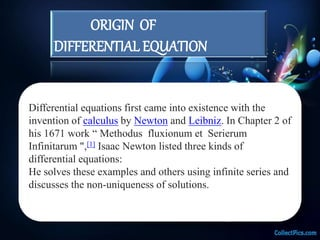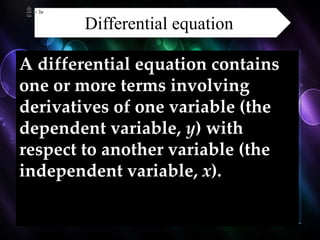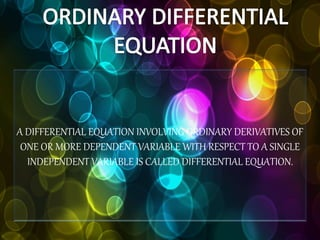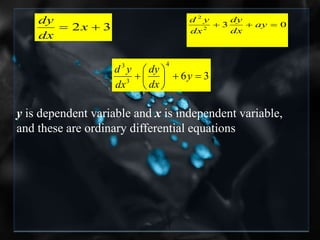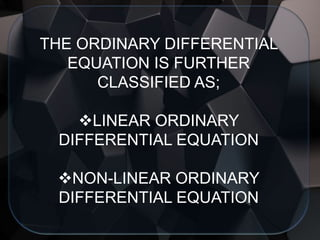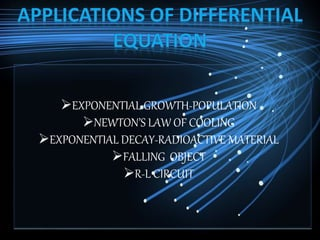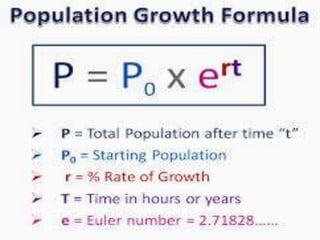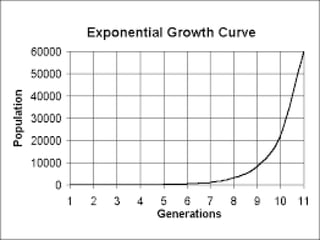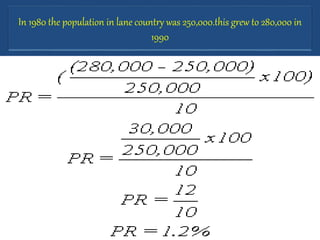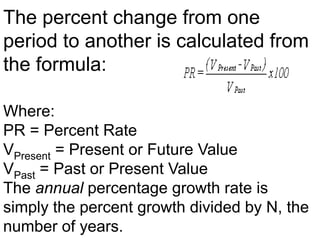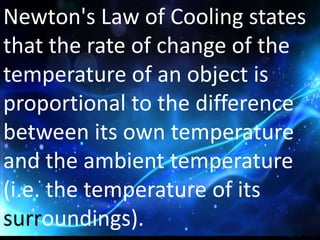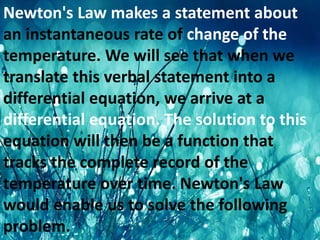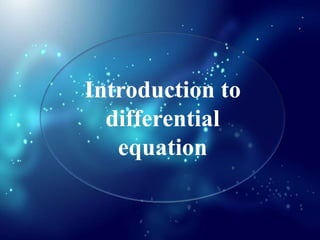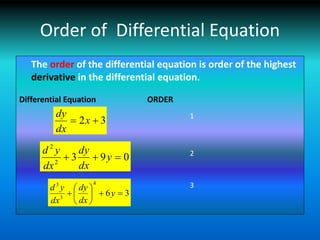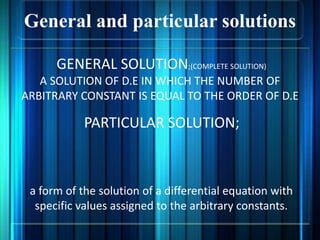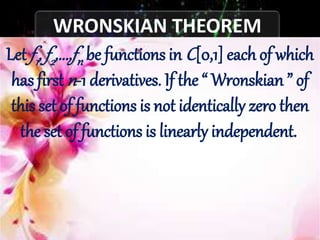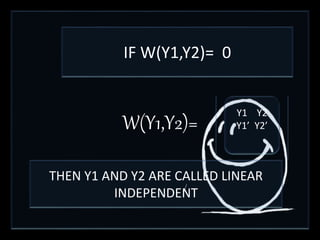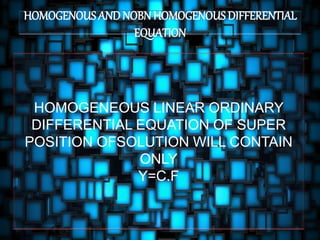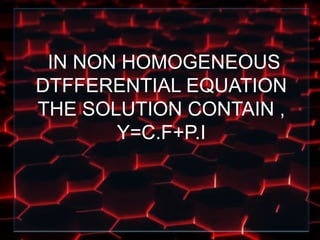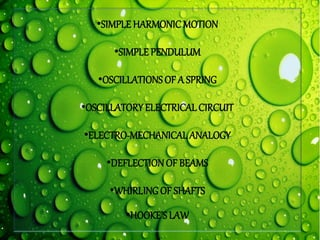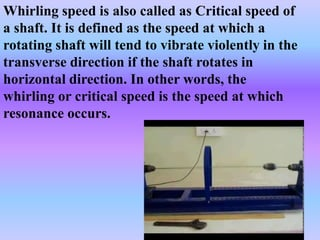1 sur 28

### honey jose presentation

• 2.  THE TERM DIFFERENTIAL EQUATION  ORDINARY DIFFERENTIAL EQUATION PARTIAL DIFFERENTIAL EQUATION ORIGIN \$ APPLICATION OF DIFFERENTIAL EQUATION
• 3. ORIGIN OF DIFFERENTIAL EQUATION Differential equations first came into existence with the invention of calculus by Newton and Leibniz. In Chapter 2 of his 1671 work “ Methodus fluxionum et Serierum Infinitarum ", Isaac Newton listed three kinds of differential equations: He solves these examples and others using infinite series and discusses the non-uniqueness of solutions.
• 4. Differential equation A differential equation contains one or more terms involving derivatives of one variable (the dependent variable, y) with respect to another variable (the independent variable, x).
• 5. A DIFFERENTIAL EQUATION INVOLVING ORDINARY DERIVATIVES OF ONE OR MORE DEPENDENT VARIABLE WITH RESPECT TO A SINGLE INDEPENDENT VARIABLE IS CALLED DIFFERENTIAL EQUATION.
• 6. y is dependent variable and x is independent variable, and these are ordinary differential equations 32  x dx dy 032 2  ay dx dy dx yd 36 4 3 3        y dx dy dx yd
• 7. THE ORDINARY DIFFERENTIAL EQUATION IS FURTHER CLASSIFIED AS; LINEAR ORDINARY DIFFERENTIAL EQUATION NON-LINEAR ORDINARY DIFFERENTIAL EQUATION
• 8. EXPONENTIAL GROWTH-POPULATION NEWTON’S LAW OF COOLING EXPONENTIAL DECAY-RADIOACTIVE MATERIAL FALLING OBJECT R-L CIRCUIT
• 11. In 1980 the population in lane country was 250,000.this grew to 280,000 in 1990
• 12. The percent change from one period to another is calculated from the formula: Where: PR = Percent Rate VPresent = Present or Future Value VPast = Past or Present Value The annual percentage growth rate is simply the percent growth divided by N, the number of years.
• 13. Newton's Law of Cooling states that the rate of change of the temperature of an object is proportional to the difference between its own temperature and the ambient temperature (i.e. the temperature of its surroundings).
• 14. Newton's Law makes a statement about an instantaneous rate of change of the temperature. We will see that when we translate this verbal statement into a differential equation, we arrive at a differential equation. The solution to this equation will then be a function that tracks the complete record of the temperature over time. Newton's Law would enable us to solve the following problem.
• 17. Order of Differential Equation The order of the differential equation is order of the highest derivative in the differential equation. Differential Equation ORDER 32  x dx dy 0932 2  y dx dy dx yd 36 4 3 3        y dx dy dx yd 1 2 3
• 18. Degree of Differential Equation Differential Equation Degree 032 2  ay dx dy dx yd 36 4 3 3        y dx dy dx yd 03 53 2 2             dx dy dx yd 1 1 3 The degree of a differential equation is power of the highest order derivative term in the differential equation.
• 19. General and particular solutions GENERAL SOLUTION;(COMPLETE SOLUTION) A SOLUTION OF D.E IN WHICH THE NUMBER OF ARBITRARY CONSTANT IS EQUAL TO THE ORDER OF D.E PARTICULAR SOLUTION; a form of the solution of a differential equation with specific values assigned to the arbitrary constants.
• 20. WRONSKIAN THEOREM Let f1, f2,...,fn be functions in C[0,1] each of which has first n-1 derivatives. If the “ Wronskian ” of this set of functions is not identically zero then the set of functions is linearly independent.
• 21. W(Y1,Y2)= Y1 Y2 Y1’ Y2’ IF W(Y1,Y2)= 0 THEN Y1 AND Y2 ARE CALLED LINEAR INDEPENDENT
• 22. HOMOGENOUS AND NOBN HOMOGENOUS DIFFERENTIAL EQUATION HOMOGENEOUS LINEAR ORDINARY DIFFERENTIAL EQUATION OF SUPER POSITION OFSOLUTION WILL CONTAIN ONLY Y=C.F
• 23. IN NON HOMOGENEOUS DTFFERENTIAL EQUATION THE SOLUTION CONTAIN , Y=C.F+P.I
• 24. SUPER POSITION PRINCIPLE IT STATES THAT,WE CAN OBTAIN FURTHER SOLUTIONS FROM GIVEN ONES BY ADDING THEM OR BY MULTIPLY THEM WITH ANY CONSTANT
• 26. •SIMPLE HARMONICMOTION •SIMPLE PENDULUM •OSCILLATIONSOF A SPRING •OSCILLATORY ELECTRICALCIRCUIT •ELECTRO-MECHANICALANALOGY •DEFLECTIONOF BEAMS •WHIRLING OF SHAFTS •HOOKE’SLAW
• 27. Whirling speed is also called as Critical speed of a shaft. It is defined as the speed at which a rotating shaft will tend to vibrate violently in the transverse direction if the shaft rotates in horizontal direction. In other words, the whirling or critical speed is the speed at which resonance occurs.